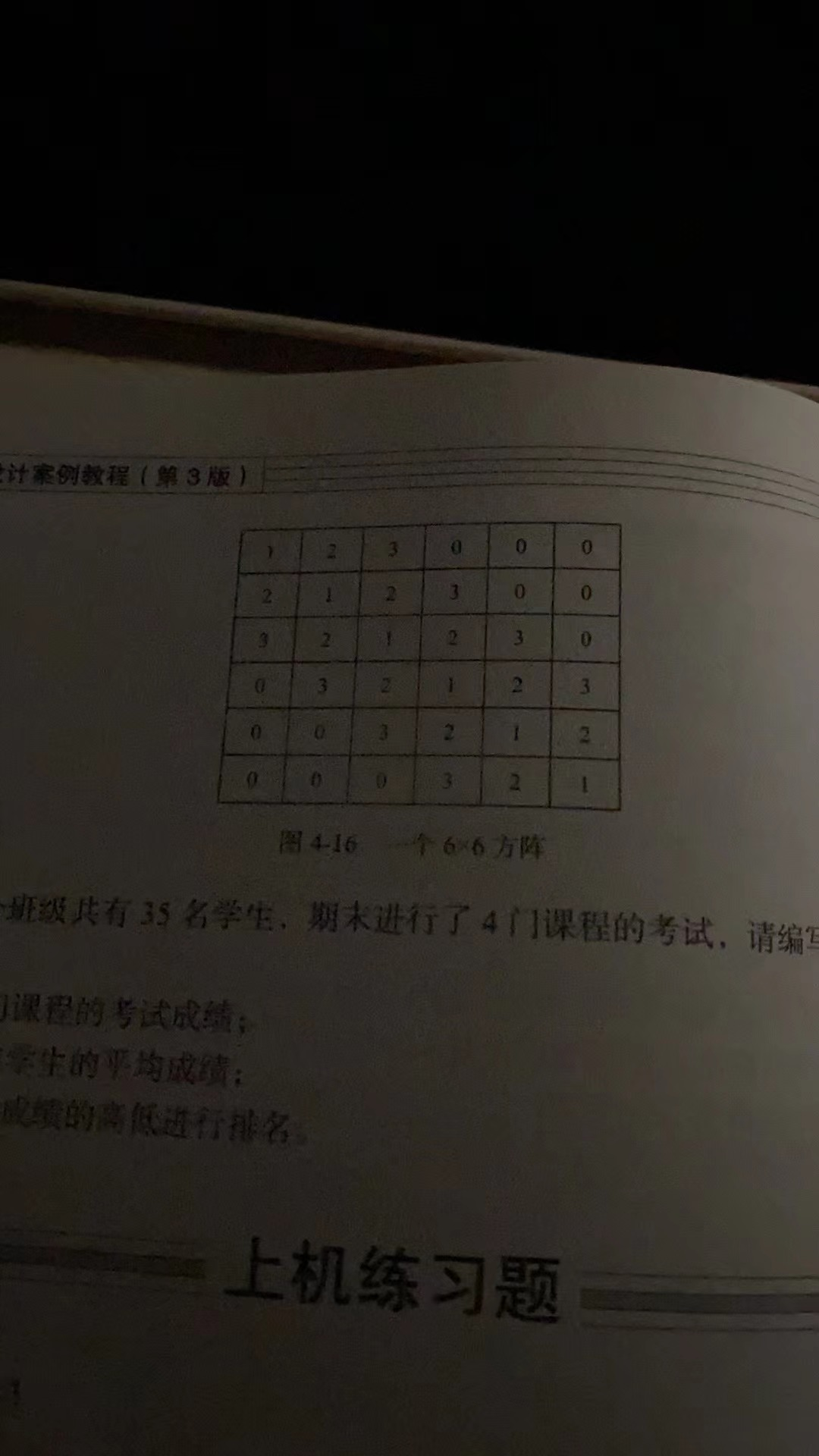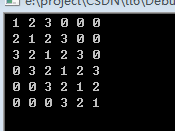2021-11-04 00:55

深夜还在学 实在是没有学明白 找一个范例图片转代码服务由CSDN问答提供 功能建议

图416 166方

生的平均成绩
的离低进行排名
上机练习题
• 写回答
• 好问题 提建议
• 追加酬金
• 关注问题
• 收藏
• 邀请回答

2条回答默认 最新

•已采纳

规律是：主对角线上的元素都是1，然后主对角线两侧数据递增，当数据大于3以后，数据为0
运行效果如下：代码：

#include <stdio.h>
int main()
{
int i,j;
int a;
for (i=0;i<6;i++)
{
for (j =0;j<6;j++)
{
if(i==j) a[i][j] = 1;//对角线元素是1
else if(j<i)
{
a[i][j] = 1 + i-j;
if(a[i][j]>3) a[i][j] =0;
}else
{
a[i][j] = 1 + j-i;
if(a[i][j]>3) a[i][j] =0;
}
}
}
//显示
for (i=0;i<6;i++)
{
for (j=0;j<6;j++)
{
printf("%2d",a[i][j]);
}
printf("\n");
}
return 0;
}

评论
解决 1 无用
打赏 举报